# insertObjectAnnotation

Annotate truecolor or grayscale image or video

## Syntax

``RGB = insertObjectAnnotation(I,shape,position,label)``
``___ = insertObjectAnnotation(___,Name=Value)``

## Description

example

````RGB = insertObjectAnnotation(I,shape,position,label)` annotates a grayscale or truecolor image with the specified `shape` and `label` at the specified `position`. The function returns a truecolor image.```

example

````___ = insertObjectAnnotation(___,Name=Value)` specifies options using one or more name-value arguments in addition to the previous syntax. For example, `FontSize=18` sets the annotation font size to `18`.```

## Examples

collapse all

Read an image into the workspace.

`I = imread("board.tif");`

Create confidence value labels using floating point numbers.

```label_str = cell(3,1); conf_val = [85.212 98.76 78.342]; for ii = 1:3 label_str{ii} = ['Confidence: ' num2str(conf_val(ii), '%0.2f') '%']; end```

Set the positions for the rectangular bounding boxes associated with the labels using the form [x y width height].

`position = [23 373 60 66; 35 185 77 81; 77 107 59 26];`

Insert the labels into the image.

`RGB = insertObjectAnnotation(I,"rectangle",position,label_str,TextBoxOpacity=0.9,FontSize=18);`

Display the annotated image.

```figure imshow(RGB) title("Annotated chips")```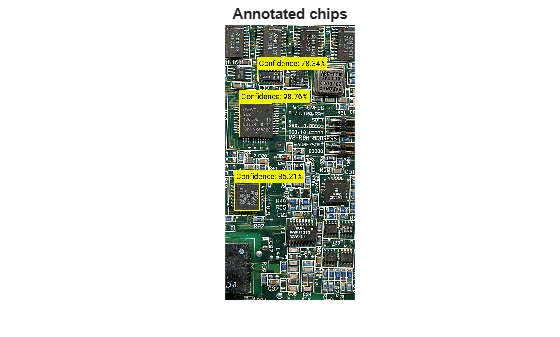Read an image into the workspace.

`I = imread("coins.png");`

Set the positions on the image for the circular bounding boxes associated with the labels. The first two values of each row specify the center coordinates of a circle, (x,y) and the third value is the radius.

`position = [96 146 31; 236 173 26];`

Set the values for the labels to display as 5 and 10, in integers. These values represent US currency in the form of a nickle and dime, respectively.

`label = [5 10];`

Insert the annotations into the image.

`RGB = insertObjectAnnotation(I,"circle",position,label,LineWidth=3,AnnotationColor=["cyan","yellow"],FontColor="black");`

Display the annotated image.

```figure imshow(RGB) title("Annotated Coins")```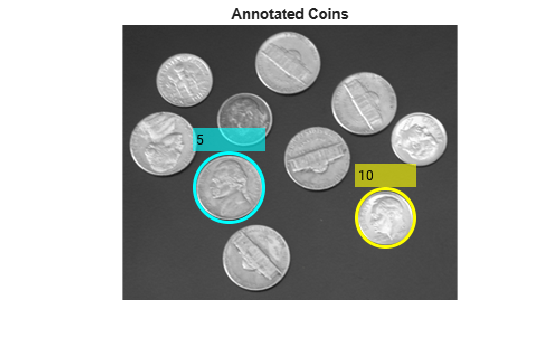## Input Arguments

collapse all

Input image, specified as an M-by-N-by-3 truecolor or an M-by-N grayscale image.

Data Types: `single` | `double` | `int16` | `uint8` | `uint16`

Shape of the annotation, specified as `"rectangle"`, `"circle"`, or `"projected-cuboid"`. If you specify a `"rectangle"` annotation be specify the position of either an axis-aligned or rotated rectangle.

Data Types: `char` | `string`

Position of the shape, specified according to the type of shape, as described in the table.

ShapePositionExample

`rectangle`

For one or more axis-aligned rectangles or filled rectangles, specify as an M-by-4 numeric matrix, where each row specifies a rectangle of the form $\left[\begin{array}{cccc}x& y& width& height\end{array}\right]$.

• M is the number of axis-aligned rectangles.

• x and `y` specify the upper-left corner of the rectangle.

• w specifies the width of the rectangle, which is its length along the x-axis.

• h specifies the height of the rectangle, which is its length along the y-axis.

`$\left[\begin{array}{cccc}{x}_{1}& {y}_{1}& widt{h}_{1}& heigh{t}_{1}\\ {x}_{2}& {y}_{2}& widt{h}_{2}& heigh{t}_{2}\\ ⋮& ⋮& ⋮& ⋮\\ {x}_{M}& {y}_{M}& widt{h}_{M}& heigh{t}_{M}\end{array}\right]$`For one or more rotated rectangles, specify in spatial coordinates as an M-by-5 numeric matrix, where each row specifies a rotated rectangle of the form [xctr yctr w h yaw].

• M is the number of rotated rectangles.

• xctr and yctr specify the center of the rectangle.

• w specifies the width of the rectangle, which is its length along the x-axis before rotation.

• h specifies the height of the rectangle, which is its length along the y-axis before rotation.

• yaw specifies the rotation angle in degrees. The rotation is clockwise-positive around the center of the rectangle.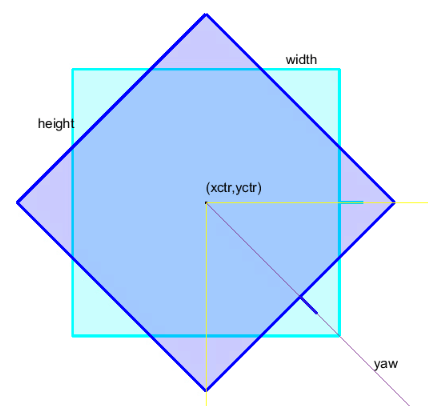`circle`

For one or more circles, specify spatial coordinates as an M-by-3 numeric matrix, where each row specifies a circle of the form [xctr yctr radius].

• M is the number of circles.

• xctr and yctr specify the center of the circle.

`$\left[\begin{array}{ccc}xct{r}_{1}& yct{r}_{1}& radiu{s}_{1}\\ xct{r}_{2}& yct{r}_{2}& radiu{s}_{2}\\ ⋮& ⋮& ⋮\\ xct{r}_{M}& yct{r}_{M}& radiu{s}_{M}\end{array}\right]$`` projected-cuboid`

For one or more projected cuboids, specify in spatial coordinates as an 8-by-2-by-M array or an M-by-8 matrix, where M is the number of projected cuboids.

When specified as an 8-by-2-by-M array, each row must contain the [x y] location of a projected cuboid vertex. The vertices connect to form a cuboid with six faces. The order of the input vertices must match the order shown in the diagram.

When specified as an M-by-8 matrix, each row specifies the dimensions of the front-facing and rear-facing sides of a projected cuboid in the form, [x1 y1 w1 h1 x2 y2 w2 h2], where [x1 y1] and [x2 y2] specify the upper-left coordinates of the front-facing and rear-facing sides, respectively, and [w1 h1] and [w2 h2] specify the corresponding widths and heights.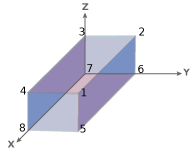Data Types: `single` | `double` | `cell` | `int8` | `int16` | `int32` | `int64` | `uint8` | `uint16` | `uint32` | `uint64`

Label to associate with a shape, specified as an M-element numeric vector, string array, vector of categorical labels, or a cell array of ASCII character vectors. If you use character vectors, string scalars, or categorical labels, you must encode them as ASCII characters. If you specify a cell array, it must be equal in length to the number of specified shape positions. You can specify a single label for all shapes using a numeric scalar, string scalar, or scalar categorical label.

Example: `[5 10]` marks the first shape with the label `5`, and the second shape with the label `10`.

### Name-Value Arguments

Specify optional pairs of arguments as `Name1=Value1,...,NameN=ValueN`, where `Name` is the argument name and `Value` is the corresponding value. Name-value arguments must appear after other arguments, but the order of the pairs does not matter.

Example: `insertObjectAnnotation(I,"rectangle",position,label,FontSize=18);` sets the font size to use for inserting annotations to `18`.

Before R2021a, use commas to separate each name and value, and enclose `Name` in quotes.

Example: `insertObjectAnnotation(I,"rectangle",position,label,"FontSize","18");` sets the font size to use for inserting annotations to `18`.

Font face of the label text, specified as a character vector or string scalar. The font face must be one of the available truetype fonts installed on your system. To get a list of available fonts on your system, use the `listTrueTypeFonts` function from the MATLAB® command prompt.

Data Types: `char` | `string`

Label text font size, specified as an integer that corresponds to points in the range of [`8 72`].

Data Types: `double` | `single` | `int8` | `int16` | `int32` | `int64` | `uint8` | `uint16` | `uint32` | `uint64`

Shape border line width, specified as a positive integer, in pixels.

Annotation color, specified as a short color name, color name, vector of color names, three-column matrix of RGB triplets.

The supported colors table lists RGB intensities in the range [0, 1], but you must specify RGB triplets in the range of your selected data type. For example, if specifying this argument as a matrix of `uint8` values, you must convert each intensity value to the range [0, 255]. To convert the listed intensity values to a `uint8` data type, use the code `uint8(255*intensity)`, where intensity is an RGB triplet value listed in the table.

You can specify a different color for each marker or one color for all markers. To specify one color for all markers, specify `AnnotationColor` as a color name or an [R G B] vector.

SpecificationFormatExample
Specify one color for all shapes (or markers)

Short color name or color name

`"r"`

`"red"`

RGB triplet

`[1 0 0]`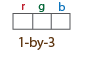Specify a color for each shape (or marker)

Vector of color names

`["red","yellow","blue"]`

Three-column matrix of RGB triplets

```[1 0 0 0 1 1 1 0 1 1 1 1]```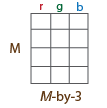Supported colors are listed in the table.

Color NameShort NameRGB TripletAppearance
`"red"``"r"``[1 0 0]``"green"``"g"``[0 1 0]``"blue"``"b"``[0 0 1]``"cyan"` `"c"``[0 1 1]``"magenta"``"m"``[1 0 1]``"yellow"``"y"``[1 1 0]``"black"``"k"``[0 0 0]``"white"``"w"``[1 1 1]`Data Types: `cell` | `char` | `uint8` | `uint16` | `int16` | `double` | `single`

Font color, specified as a short color name, color name, vector of color names, three-column matrix of RGB triplets.

The supported colors table lists RGB intensities in the range [0, 1], but you must specify RGB triplets in the range of your selected data type. For example, if specifying this argument as a matrix of `uint8` values, you must convert each intensity value to the range [0, 255]. To convert the listed intensity values to a `uint8` data type, use the code `uint8(255*intensity)`, where intensity is an RGB triplet value listed in the table.

You can specify a different color for each font string or one color for all strings. To specify one color for all strings, specify `FontColor` as a color name or an [R G B] vector.

Data Types: `logical` | `uint8` | `uint16` | `int16` | `double` | `single`

Opacity of the text label box background, specified as a scalar in the range of ```[0, 1]```. A value of `0` renders the background of the label text box as fully transparent, while a value of `1` renders it as fully opaque.

Data Types: `double` | `single` | `int8` | `int16` | `int32` | `int64` | `uint8` | `uint16` | `uint32` | `uint64`

Original orientation indicator, specified as a numeric or logical `1` (`true`) or `0` (`false`). A value of `1` (`true`) displays the original orientation of the rotated rectangle by using an arrow annotation on the bounding box. The original orientation is defined as a zero rotation angle. A value of `0` (`false`) does not show the arrow annotation. The `ShowOrientation` name-value argument applies only to rotated rectangles.

Data Types: `logical` | `integer`

## Output Arguments

collapse all

Output image, returned as an M-by-N-by-3 truecolor image.

## Version History

Introduced in R2012b

expand all